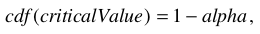Test operations analyze the input data to examine the validity of a specific hypothesis. Common tests involves a computation of some numeric value, also known as "test statistic", which is usually compared with a critical value in order to determine if you should accept or reject the test hypothesis (H0). Most tests compute a critical value for the given significance alpha (default value 0.05 or specified via the /ALPH flag). Some tests compute the P-value which you can then directly compare to the desired significance value.

Critical values have been traditionally published in tables for various significance levels and tails of distributions. They are by far the most difficult technical aspect in implementating statistical tests. Critical values are usually obtained from the inverse of the CDF for the appropriate distribution function, i.e., by solving the equationwhere alpha is the significance. In some distributions (e.g., Friedman's) the calculation of the CDF is so computationally intensive that it can be impractical to compute for very large parameters. Fortunately, when parameters become large one can usually find an adequate approximate solution. Whenever possible Igor's tests provide exact critical values as well as the common relevant approximations.

Comparison of critical values with published table values can sometimes be interesting as there does not appear to be a standard for determining the critical value when the CDF takes a finite number of discreet values (step-like). In this case the CDF most likely attains the value (1-alpha) in a vertical transition so one could use the x-value for the vertical transition as a critical value or the x-value of the subsequent vertical transition. Some tables reflect a "convervative" approach and print the x-value of subsequent transitions.

Statistical test operations can print some of their results to the history window and save them in a wave in the current data folder. Result waves have a fixed name associated with the operation. Elements in the wave are designated by dimension labels. You can use the /T flag to display the results of the operation in a table with dimension labels. The argument for this flag determines what happens when you kill the table. You can use the /Q in all test operations to restrict printing information in the history window and you can use the /Z flag to make sure that the operations do not report errors except by setting the V_Flag variable to -1.

Statistical test operations tend to include several variations of the named test. You can usually choose to execute one or more variations by specifying the appropriate flags. The following table can be used as a guide for identifying the operation associated with a given test name.

Statistical TestsForumSupportGallery